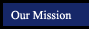Algebra 1 - Concepts, Skills and Problem Solving
Analyzing Linear Equations
Chapter 4
 Section Title Notebook files More examples (in pop-up window) 4.1 Rate of Change and Slope new per4 per6 notebook express 4.2 Slope and Direct Variation new per4 per6 notebook express 4.3 Graphing Equations in Slope-Intercept Form new per4 per6 notebook express 4.4 Writing Equations in Slope-Intercept Form new per4 per6 notebook express 4.5 Writing Equations in Point-Slope Form new per4 per6 notebook express 4.6 Statistics: Scatter Plots and Lines of Fit 4.7 Geometry: Parallel and Perpendicular Lines new per4 per6 notebook express

Online Scientific Calculator from Calculator.com

Math Glossary
(more sophisticated than it looks!)